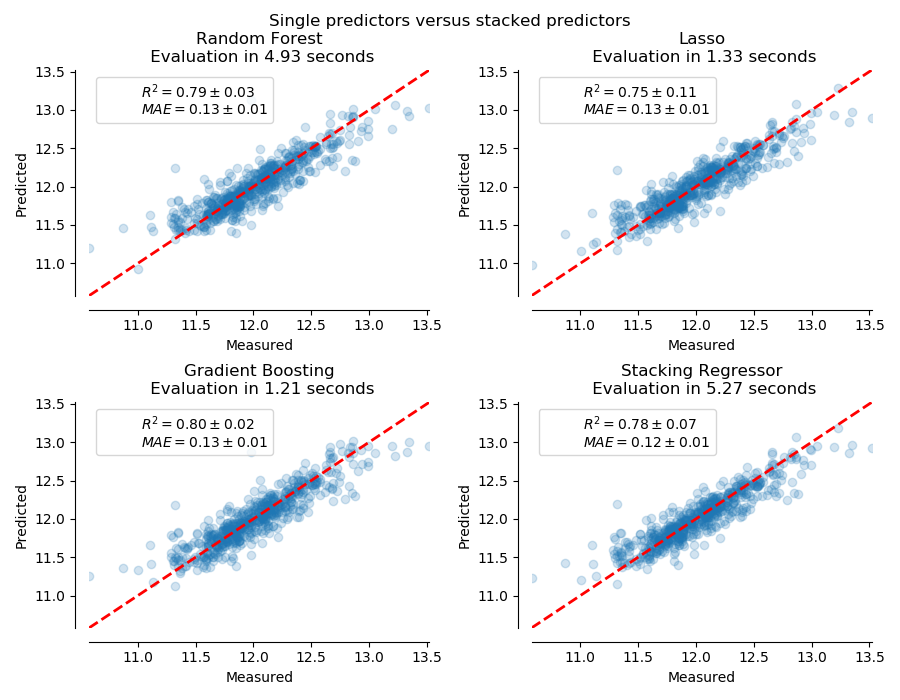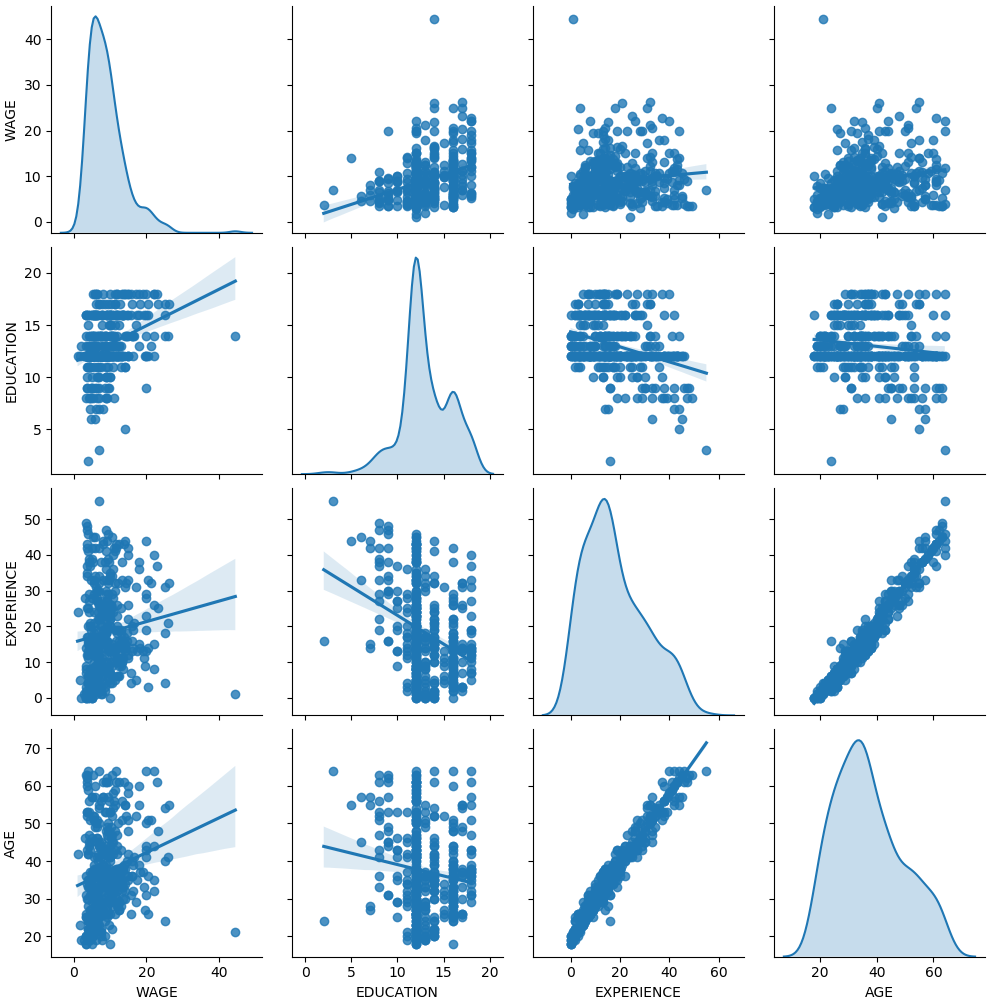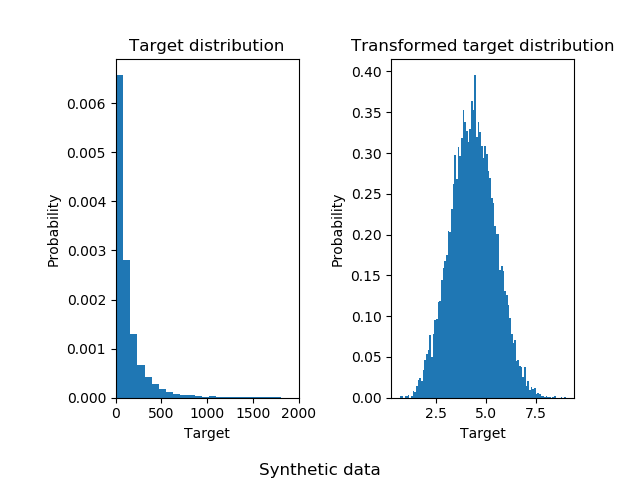# sklearn.linear_model.RidgeCV¶

class sklearn.linear_model.RidgeCV(alphas=(0.1, 1.0, 10.0), *, fit_intercept=True, normalize=False, scoring=None, cv=None, gcv_mode=None, store_cv_values=False)

alphas ndarray of shape (n_alphas,), default=(0.1, 1.0, 10.0)

fit_intercept bool, default=True

normalize bool, default=False
fit_intercept设置为False 时，将忽略此参数。如果为True，则在回归之前通过减去均值并除以l2-范数来对回归变量X进行归一化。如果你希望标准化，请先使用 sklearn.preprocessing.StandardScaler，然后调用fit 估算器并设置normalize=False
scoring string, callable, default=None

cv int, cross-validation generator or an iterable, default=None

- None，使用有效的“留一法”交叉验证（也称为通用交叉验证）
- 整数，用于指定折叠数。
- CV分割器
- 可迭代生成(分割训练、测试)索引数组。

gcv_mode {‘auto’, ‘svd’, eigen’}, default=’auto’

'svd' : force use of singular value decomposition of X when X is dense, eigenvalue decomposition of X^T.X when X is sparse.
'eigen' : force computation via eigendecomposition of X.X^T<br />
“auto”模式是默认模式，用于根据训练数据的形状选择两者中更合适的选项。
store_cv_values bool, default=False

cv_values_ ndarray of shape (n_samples, n_alphas) or shape (n_samples, n_targets, n_alphas), optional

coef_ ndarray of shape (1, n_features) or (n_classes, n_features)

intercept_ float or ndarray of shape (n_targets,)

alpha_ float

best_score_ float

Ridge

RidgeClassifier

RidgeClassifierCV

>>> from sklearn.datasets import load_diabetes>>> from sklearn.linear_model import RidgeCV>>> X, y = load_diabetes(return_X_y=True)>>> clf = RidgeCV(alphas=[1e-3, 1e-2, 1e-1, 1]).fit(X, y)>>> clf.score(X, y)0.5166...

fit(X, y[, sample_weight]) 拟合带有交叉验证的Ridge回归模型。
get_params([deep]) 获取此估计器的参数。
predict(X) 使用线性模型进行预测。
score(X, y[, sample_weight]) 返回预测的确定系数R ^ 2。
set_params(**params) 设置此估计器的参数。
__init__(alphas=(0.1, 1.0, 10.0), *, fit_intercept=True, normalize=False, scoring=None, cv=None, gcv_mode=None, store_cv_values=False)

[源码]

fit（X，y，sample_weight = None ）

[源码]

X ndarray of shape (n_samples, n_features)

y ndarray of shape (n_samples,)

sample_weight float or ndarray of shape (n_samples,), default=None

self object

get_params(deep=True)

[源码]

deep bool, default=True

params mapping of string to any

predict(X)

[源码]

X array_like or sparse matrix, shape (n_samples, n_features)

C array, shape (n_samples,)

score(X, y, sample_weight=None)

[源码]

X array-like of shape (n_samples, n_features)

y array-like of shape (n_samples,) or (n_samples, n_outputs)
X的真实值。
sample_weight array-like of shape (n_samples,), default=None

score float

set_params(**params)

[源码]

**params dict

self object

## sklearn.linear_model.RidgeCV使用示例¶